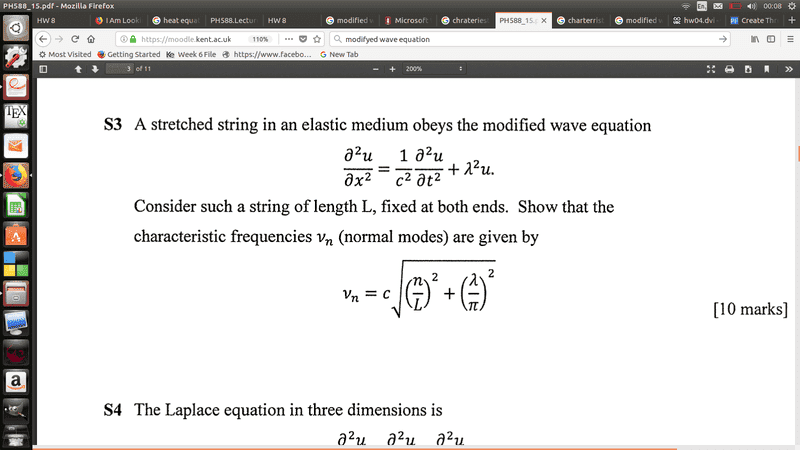# PDE modifed wave equation

Taylor_1989

## Homework StatementI am having a issue understanding this question I have solve the PDE below, but I cant understand where or how you the characteristic frequency, what more confusing is that I don’t know if that lambda is just a constant or a wavelength.

## The Attempt at a Solution

$$U\left(x,t\right)=X\left(x\right)T\left(t\right)$$

$$\frac{X''\left(x\right)}{X\left(x\right)}=\frac{T''\left(t\right)}{c^2T}+\lambda ^2=-k^2$$

$$X''\left(x\right)+k^2X\left(x\right)=0$$

using boundary condtions ##U\left(0,t\right)=U\left(l,t\right)=0##, ##X(x)## can be forund to be:

$$X\left(x\right)=C_1sin\left(kx\right),\:X_n\left(x\right)=C_nsin\left(\frac{\pi n}{l}\right)$$

Now solving for T

$$T''\left(t\right)+\left(k^2+\lambda ^2\right)c^2T\left(t\right)=0$$

$$b^2=\left(c^2\left(\lambda ^2+k^2\right)\right)$$

$$T''\left(t\right)+b^2T\left(t\right)=0$$

Solving this I get the following equation

$$T\left(t\right)=C_2cos\left(bt\right)+C_3sin\left(bt\right),\:T_n\left(t\right)=A_ncos\left(c\sqrt{\lambda ^2+k^2}\right)+B_nsin\left(c\sqrt{\lambda \:^2+k^2}\right)$$

$$U_n\left(x,t\right)=\sum \:sin\left(\frac{\pi n}{l}\right)\left(A_ncos\left(c\sqrt{\lambda \:^2+k^2}t\right)+B_nsin\left(c\sqrt{\lambda \:\:^2+k^2}t\right)\right)$$

and this is where I am lost how do I find the characteristic frequency from this? I just cant seem to understand how they have gotten the follwing euqation,

I know that normally the frequency and be workout from ##f_n=\frac{nc}{2l}## where c is the speed of the wave along the string.

Could anyone please give some adice it would be much appreciated

edit: I just thought of something which give me somewhat of the correct answer. But I am not too sure if I can say c is a constant if it is I have the correct ans, but as I mentioned perivous I assume c is the speed of the wave.

Here what I have.

If I have a standing wave then, I have two solutions

$$y_1=Asin\left(kx-\omega t\right)$$

and

$$y_2=Asin\left(kx+\omega t\right)$$

as this sinerio gives a standing wave then I get

$$y_1+y_2=2Asin\left(kx\right)cos\left(\omega t\right)$$

so comparing this to what I have I can see that

$$\omega =2\pi f=c\sqrt{\lambda ^2+k^2}=c\sqrt{\lambda ^2+\left(\frac{n\pi }{L}\right)^2}$$

If I reagrange and solve for ##f_n## I get the following

$$f_n=\frac{c}{2}\sqrt{\frac{\lambda ^2}{\pi ^2}+\frac{1}{\pi ^2}\left(\frac{n\pi }{L}\right)^2}$$

which simplifying to the ans required except I have a 1/2 in mine can this be absorbed by c as it a constant or is there a step I am missing?

Last edited:

## Answers and Replies

$$X\left(x\right)=C_1sin\left(kx\right),\:X_n\left(x\right)=C_nsin\left(\frac{\pi n}{l}\right)$$